American Journal of Modern Physics
Volume 4, Issue 4, July 2015, Pages: 189-195

Subatomic Particle Structures and Unified Field Theory Based on Yangton and Yington Hypothetical Theory

Edward T. H. Wu

Solar Buster Corporation, Los Angeles, USA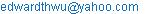Edward T. H. Wu. Subatomic Particle Structures and Unified Field Theory Based on Yangton and Yington Hypothetical Theory. American Journal of Modern Physics. Vol. 4, No. 4, 2015, pp. 189-195. doi: 10.11648/j.ajmp.20150404.15

Abstract: A hypothetical theory of a Yangton and Yington circulating pair with an inter-attractive "Force of Creation" is proposed as the "Origin of Creation". When this circulating pair travels in the space it is known as "Photon". Otherwise, at still, it is known as "Wu’s Particles" also as "Still Photon", which makes the basic building block of all matters. The structures of Quarks, Neutrinos, Higgs Boson particles, Electron, Positron, Proton, Neutron, and Dark Matters are proposed based on "Wu’s Particles". Also, it is assumed that "Force of Creation" is the only fundamental force in the universe that could create and unify the Four Basic Forces. Gravitation is formed by the attractive force between two Higgs Boson particles with String Structures that are made of "Wu’s Particles" of the same circulation direction. Electric Forces, either attractive or repulsive, can be formed between static Electrons and Protons/Positrons. Electromagnetic Force is generated between moving electrons and positrons/Protons. Two atoms both with single outer layer Electron are attractive to each other while circulating and spinning in the same direction and repulsive in the opposite directions. Both the Weak Force between Neutron and Positron as well as the Strong Force between two Neutrons also between Proton and Neutron are proposed and interpreted based on “Wu’s Particles” and “Force of Creation”.

Keywords: Yangton, Yington, Wu’s Particles, Photon, Electron, Proton, Neutron, Unified Field Theory

1. Introduction

It is proposed by Edward T. H. Wu, a hypothetical theory (Ref. 1) that a pair of super fine particles, Yangton and Yington, with an inter-attractive "Force of Creation", can be spontaneously created at anywhere and anytime in the universe as the "Origin of Creation" that could make "Something from Nothing". Meantime, because of the reversible process enforced by the inter-attractive "Force of Creation", after formation, within no time, Yangton and Yington recombine themselves to destroy each other so as to ensure that "Something Becomes Nothing" and the whole universe returns back to an empty space.

In the beginning, there was nothing in the universe until the Big Bang (Ref. 2) explosion. It is proposed that Photon (Ref. 3) was first formed by a pair of Yangton and Yington particles; however instead of recombination and destroy of each other, induced by the explosion; they started moving toward each other permanently in a circular orbit. Free Photon travels at light speed which can combine Particle Physics (Ref. 4) and Quantum Mechanics (Ref. 5) to explain a variety of properties of light (Ref. 1). Still Photon, also known as "Wu’s Particles", on the other hand forms the building block of all Subatomic Particles including Quarks (Ref. 6), Neutrinos (Ref. 7), Higgs Boson particles (Ref. 8), Electron (Ref. 9), Positron (Ref. 10), Proton (Ref. 11), Neutron (Ref. 12) and Dark Matters (Ref. 13), which will be explained in detail in this paper.

Furthermore, it is assumed that "Force of Creation" is the only fundamental force in the universe that can create and unify the Four Basic Forces (Ref. 14). Gravitation (Ref. 15) is induced by the attractive force between two Higgs Boson particles with String Structures (Ref. 16) that are made of "Wu’s Particles" of the same circulation direction. Electric Forces, either attractive or repulsive, can be formed between static Electrons and Protons/Positrons (Fig. 5). Electromagnetic Force (Ref. 17) is generated between moving electrons and positrons/Protons. Two atoms both with single outer layer electrons can form attractive force between each other with electron circulating and spinning in the same direction and repulsive force while in the opposite directions.

2. The Whole Universe is Built on Yongton and Yington Circulating Pair

It is proposed that a pair of super fine particles, Yangton and Yington (Fig. 1) with an inter-attractive "Force of Creation", can be spontaneously formed in the universe at anytime and anywhere. However, due to the enforcement of the inter-attractive "Force of Creation", Yangton and Yington immediately recombine and destroy each other such that something becomes nothing and everything disappeared again in the empty space.

Not until the Big Bang explosion, Yangton and Yington pairs became permanently exist by absorbing "Energy of Formation" and started moving simultaneously toward each other in a circular orbit against the inter-attractive "Force of Creation" (Fig. 2). These Yangton and Yington circulating pairs are known as Photons. A "Free Photon" can travel in different matters at different speeds. A "Still Photon" also named as "Wu’s Particles", on the other hand is the basic building block of all matters.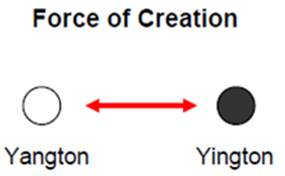Fig. 1. A hypothetical Yangton and Yington pair and Force of Creation.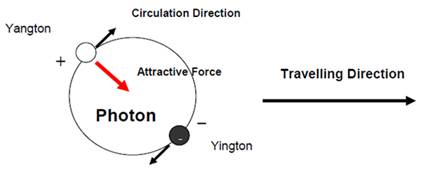Fig. 2. A hypothetical Photon.

3. Free Photon is a Particle and Also a Wave

Because of the inter-attractive force between Yangton and Yington, it is assumed that Yangton carries one positive unit-charge and Yington carries one negative unit-charge and together they form an electric dipole. These unit-charges are the basic units of the normal electric charges that are carried by Electrons and Protons except in a much smaller scale.

Resulting from the circulation of the Yangton and Yington pair, or in another word the rotation of the electric dipole inside the Photon, Electromagnetic Wave (Ref. 20) can be generated and carried by a Free Photon to far distance at light speed (Fig. 3). From a macroscopic point of view, Photon can be considered as a neutral particle, simply because any external electric force applied on Yangton can balance out completely that on Yington due to their extremely fine circulation loop.

Electron is different from Yington, because it is assumed that Electron is made of a cluster of "Wu’s Particles" (Fig. 5), which carries one negative electric charge that contains a number of negative electric "Unit-charges". Positron and Proton on the other hand are also different from Yangton in a similar way except containing positive electric "Unit-charges" (Fig. 5).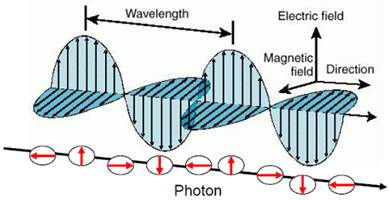Fig. 3. Electromagnetic wave of a Photon generated along traveling.

4. "Wu’s Particles" is the Building Block of All Matters

It is assumed that all matters are made of "Wu’s Particles", a "Still Photon" composed of a Yangton and Yington circulating pair confined inside a substance. With the preference of orientation due to the attractive force between Yangton and Yington particles and the dipoles in the adjacent "Wu’s Particles", unique Subatomic Particle structures can be constructed by using "Wu’s Particles" as the building block.

5. String Structure

String and Ring Structures (Fig. 4) can be built by a number of disc-like "Wu’s Particles"; each contains a pair of Yangton and Yington in a circular orbit, positioned 180 degrees away from each other of the adjacent disks in the same circulation direction. The String Structure can be used for the implementation of the String Theory (Ref. 16). Also, due to the attractive force generated between two String Structures of the same circulation direction (Fig. 4), Gravitation Force can be induced between two Higgs Boson particles.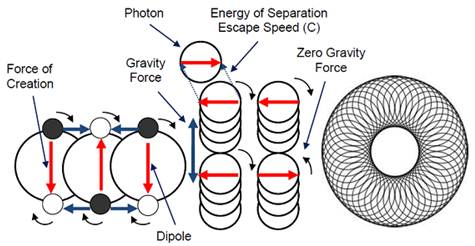Fig. 4. Wu’s Particles stack up in a preferred direction to form String and Ring structures.

6. Electron & Positron

When a multiple of "Wu’s Particles" come together, they can stack up to form string and ring structures such as that in Fig. 4, or cross lap each other’s orbits to form a structure that is either with Yingtons circulating the Yangton Center such as the Electron (Fig. 5) or with Yangtons circulating the Yington Center as the Positron (Fig 5).

In Electron, because the repulsive force between Yangtons are smaller than the "Force of Creation", the attractive force between Yangton and Yington pair, Yangtons are loosely confined in the center of Electron, which is enforced by the centrifuge force induced from the circulation of Yingtons. Similar mechanism can also apply to Positron. Since Positron is the anti-matter of Electron, when they collide to each other, both Positron and Electron are destroyed and Gamma Ray (γ) is released, this is known as Positron-Electron Annihilation (Fig. 6).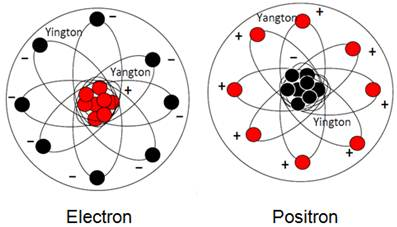Fig. 5. Hypothetical structures of Electron and Positron.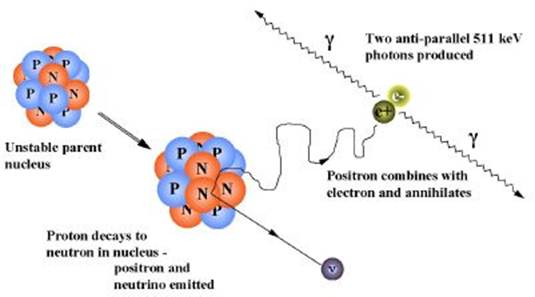Fig. 6. Proton Decays and Positron-Electron Annihilation.

7. Proton & Neutron

In the modern Standard Model of Particle Physics, the Proton is composed of three Quarks: two Up Quarks and one Down Quark (Fig. 8). The Proton has one net positive electric charge and a mass about one atomic mass unit. The Quarks of the Proton are held together by the Strong Force, mediated by Gluons.

The Neutron is also composed of three quarks: two Down Quarks and one Up Quark (Fig. 7). The Neutron has no net electric charge and a mass slightly larger than that of a Proton. Like the Proton, the Quarks of the Neutron are also held together by the Strong Force, mediated by Gluons.

Protons and Neutrons constitute the nucleus of an atom, and they are collectively referred to as nucleons. Within the nucleus, since interacting Protons have a mutual electromagnetic repulsion that is stronger than their attractive nuclear interaction, Neutrons are a necessary constituent of any atomic nucleus that contains more than one Proton. Neutrons bind with Protons and one another in the nucleus via the nuclear force, effectively moderating the repulsive forces between the Protons and stabilizing the nucleus.

8. Beta Decay

Free Neutrons are unstable, having a mean lifetime of just under 15 minutes from a radioactive decay known as Beta Decay (Ref. 21), where one of the Neutron's Quarks changes flavor via the weak interaction. Since the mass of Neutron is slightly greater than Proton, it is possible that a Neutron can decays into a Proton, an Electron, and an Electron Antineutrino. In free Neutron, Beta Decay can be denoted as:

n0 → p+ + e + νe

Where p+, e, and νe denote the Proton, Electron and Electron Antineutrino, respectively.

Free Protons are stable. The transformation of a free Proton to a Neutron plus a Positron and an Electron Neutrino is energetically impossible, because a free Neutron has a greater mass than a free Proton.

Most Neutrons within nuclei are stable. According to the nuclear shell model, the Protons and Neutrons of a nuclide are a quantum mechanical system organized into discrete energy levels with unique quantum numbers. For a Neutron to decay, the resulting Proton requires an available state at lower energy than the initial Neutron state. In stable nuclei the possible lower energy states are all filled, meaning they are each occupied by two Protons with spin up and spin down. The Pauli Exclusion Principle therefore disallows the decay of a Neutron to a Proton within stable nuclei.

9. Inverse Beta Decay

Inside a nucleus, a Proton can transform into a Neutron via Inverse Beta Decay (Fig. 6), if an energetically allowed quantum state is available for the Neutron. This transformation occurs by emission of a Positron and an Electron Neutrino:

p+ → n0 + e+ + νe

The transformation of a Proton to a Neutron inside of a nucleus is also possible through Electron capture:

p+ + e → n0 + νe

10. Structures of Neutron and Proton

Neutron is composed of three Quarks: one Up Quarks and two Down Quark. Since all matters are made of Wu’s Particles either in String or Ring structures. It is believed that a Neutron contains three string components with shape as a donut or a triangle pretzel (Fig. 7).

Proton is also composed of three Quarks: two Up Quarks and one Down Quark. Therefore, likes the Neutron, it also processes three string components with shape as a donut or a triangle pretzel. However, because of the Inverse Beta Decay, it is believed that Proton contains a Neutron with an embedded Positron and Electron Neutrino (Fig. 8).

Since Positron is the anti-matter of Electron and Electron Neutrino is the anti-matter of Electron Antineutrino, in Beta Decay, free Neutron at high energy state first incubates two pairs of antimatters: Electron/Positron pair & Electron Neutrino/Electron Antineutrino pair, then emits the Electron and Electron Antineutrino, while maintains the Neutron, Positron and Electron Neutrino to form a Proton at low energy state.

p+ → n0 + e+ + νe           (Inverse Beta Decay)

n0 = n0 + (e+ + e)+e + νe) = (n0 + e+ + νe) + e + νe

= p+ + e + νe

n0 → p+ + e + νe           (Beta Decay)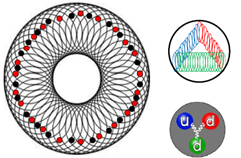Fig. 7. A hypothetical structures of Neutron.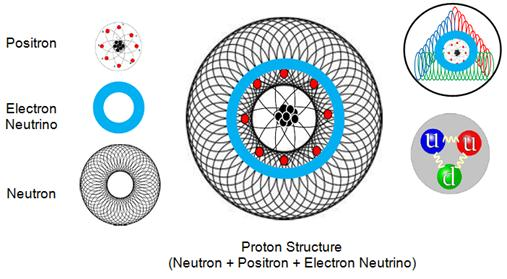Fig. 8. A hypothetical structures of Proton.

11. Dark Matter

Dark Matter is assumed to be made by a multiple of "Wu’s Particles", also known as "Still Photons", composed of Yangton and Yington circulating pairs. For example, a tetrahedral structure of four Yangton and Yington pairs (Fig. 9), where each Yangton and Yington pair is circulating on its own orbit at 109.5 degree away from the other three pairs. Because the Yangton Center is very close or even coincides with the Yington Center, there is a very small or even no dipole in the center of tetrahedral structure, therefore the attractive force between the dipoles of two adjacent Dark Matters is much smaller comparing to that of "Wu’s Particles" in the String Structures. As a result, the Gravitation Force between Dark Matter and other substance is also extremely small.

In contrary to "Wu’s Particles", without attractive force, Dark Matter can’t be used as the building block of any substances. Furthermore, because Dark Matter is an extremely small, stable and isolated particle, which can’t emit Free Photons at any temperature, therefore it is totally invisible, that is why it is known as "Dark Matter".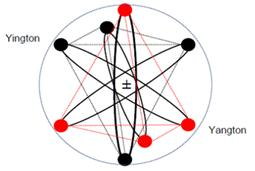Fig. 9. A hypothetical Dark Matter of tetrahedral structure.

12. Force of Creation and Four Basic Forces

It is assumed that the Four Basic Forces including Gravitation, Electromagnetic Weak and Strong Forces in the universe are generated from the "Force of Creation", the attractive force between Yangton and Yington circulating pair. Each of these forces is associated with a unique structure made of "Wu’s Particles" inside the subatomic particles.

13. Gravitation

A Higgs Boson particle with String Structure made of "Wu’s Particles" has dipoles circulating in one direction (Fig. 4) (Fig. 10). When two Higgs Boson particles come together, attractive force can be formed if the diploes of the two particles follow the same circulation direction. There is no interference between the two particles that have opposite circulation directions because of the cancellation due to the "Counter Effects". This "Attractive Force" between two Higgs Boson particles is known as Gravitation Force which is commonly found in the universe between matters.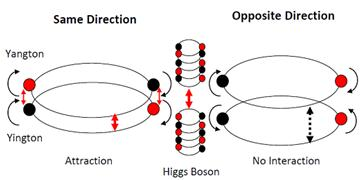Fig. 10. Gravitation force between two Higgs Boson particles.

14. Electromagnetism

In addition to the attractive and repulsive Electric Forces formed between the static Electrons and Protons/Positrons (Fig. 5), Electromagnetic Force (Ref. 17) can be generated between moving electrons and positrons/Protons. When two atoms come together with their outer layer Electrons circulating and spinning in the same direction, they prefer to stay 180 degrees away from each other so as to form attractive force between the two atoms. Otherwise, the repulsive force will be formed as the Electrons circulating and spinning in the opposite directions (Fig. 11).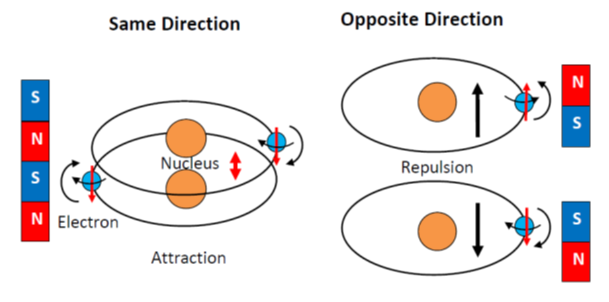Fig. 11. Electromagnetic force between two atoms with single outer layer electrons.

15. Weak Force & Strong Force

The bonding force between Neutron and Positron is known as Weak Force which is induced by the multiple Yangtons on the surface of Positron. In Inverse beta decay, the Weak Force between Neutron and Positron in a Proton is overcome by the kinetic energy of the Proton such that Proton can transfer to Neutron by emitting a Positron and an Electron Neutrino.

In order to balance the repulsive Electromagnetic Force caused between Protons, Strong Force is needed to hold Protons together in the Nucleus. Strong Force is the attractive force generated between Neutron and Neutron, and also between Neutron and Proton. When two Neutrons with String Structures made of "Wu’s Particles" come together, attractive force can be generated between the two Neutrons with either the same or opposite circulation directions. This "Attractive Force" is known as Strong Force (Fig. 12) which is many magnitudes larger than the Gravitation Force.Fig. 12. Strong Force between two Neutrons.

When a Neutron comes close to a Proton, which is made of Neutron, Positron and Electron Antineutrino, both the Weak Force between Neutron and Positron (Fig. 13), and the Strong Force between Neutron and Neutron (Fig. 13) are generated to overcome the repulsive force between Protons so as to keep them together inside the nucleus.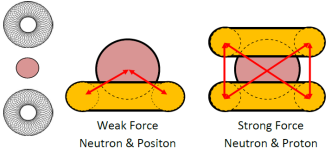Fig. 13. Weak Force and Strong Force.

16. Unified Field Theory

"Force of Creation" between Yangton and Yington pair is the fundamental force of the universe. With different structures of substances, Four Basic Forces based on "Force of Creation" can be induced and applied to everywhere in the universe which is known as the Unified Field Theory. For examples, Gravitation Force can be created between two Higgs Boson particles made of String Structures; Electromagnetic Force can be generated between Electron and Proton; Weak Forced can be formed between Neutron and Positron; Strong Force can be produced between two Neutrons, and also between Neutron and Proton.

17. Conclusion

In conclusion, a hypothetical theory of a Yangton and Yington circulating pair with an inter-attractive "Force of Creation" is proposed to form the entire universe. When this pair of magic particles travels in the space, it is known as Photon which delivers the radiation energy. When sits in still, it is known as "Wu’s Particles" or "Still Photon" which can form the building block of all matters such as Quarks, Neutrinos, Higgs Boson particles, Electron, Positrons, Proton, Neutron, and Dark Matters. Furthermore, the "Force of Creation" between the Yangton and Yington pair is the fundamental force in the universe which can induce and unify the Four Basic Forces: Gravitation, Electromagnetism, Weak and Strong Forces.

References

1. Edward T. H. Wu, "Yangton and Yington – A Hypothetical Theory of Everything", Science Journal of Physics, Volume 2015, Article ID sjp-242, 6 Pages, 2015, doi: 10.7237/sjp/242
2. "Big-bang model". Encyclopedia Britannica. Retrieved 11 February 2015.
3. http://en.wikipedia.org/wiki/Photon.
4. Braibant, S.; Giacomelli, G.; Spurio, M. (2009).Particles and Fundamental Interactions: An Introduction to Particle Physics. Springer. pp. 313–314. ISBN 978-94-007-2463-1.
5. Walter Greiner (2001).Quantum Mechanics: An Introduction. Springer. p. 29.ISBN 3-540- 67458-6.
6. "Quark (subatomic particle)". Encyclopedia Britannica. Retrieved 2008-06-29.
7. "Neutrino". Glossary for the Research Perspectives of the Max Planck Society. Max Planck Gesellschaft. Retrieved 2012-03-27.
8. http://en.wikipedia.org/wiki/Higgs_boson
9. http://en.wikipedia.org/wiki/Electron
10. P. A. M. Dirac. "The quantum theory of the Electron" (PDF).
11. Basdevant, J. L.; Rich, J.; M. Spiro (2005). Fundamentals in Nuclear Physics. Springer. p. 155. ISBN 0-387-01672-4.
12. Thomas, A.W.; Weise, W. (2001), The Structure of the Nucleon, Wiley-WCH, Berlin, ISBN 3-527-40297-7
13. Dark matter. CERN. Retrieved on 17 November 2014.
14. Davies, Paul (1986), The Forces of Nature, Cambridge Univ. Press 2nd ed.
15. Chandrasekhar, Subrahmanyan (2003). Newton's Principia for the common reader. Oxford: Oxford University Press. (pp.1–2).
16. Polchinski, Joseph (1998). String Theory, Cambridge University Press ISBN 0521672295.
17. Ravaioli, Fawwaz T. Ulaby, Eric Michielssen, Umberto (2010). Fundamentals of applied electromagnetics (6th ed.). Boston: Prentice Hall. p. 13. ISBN 978-0-13-213931-1.
18. J. Christman (2001). "The Weak Interaction" (PDF). Physnet. Michigan State University.
19. Chapter 4 NUCLEAR PROCESSES, THE STRONG FORCE, M. Ragheb 1/27/2012, University of Illinois
20. Reitz, John; Milford, Frederick; Christy, Robert (1992). Foundations of Electromagnetic Theory (4th Ed.). Addison Wesley. ISBN 0-201-52624-7.
21. Konya, J.; Nagy, N. M. (2012). Nuclear and Radiochemistry. Elsevier. pp. 74-75. ISBN978-0-12-391487-3.

 Contents 1. 2. 3. 4. 5. 6. 7. 8. 9. 10. 11. 12. 13. 14. 15. 16. 17.
Article ToolsAbstractPDF(1970K)